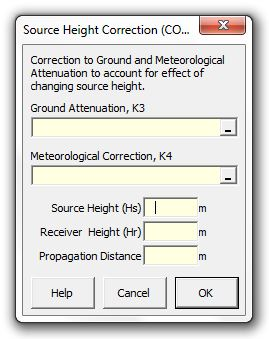### Strutt Help

Source Height Correction (CONCAWE)    1/1

Strutt|Environmental Noise|CONCAWE Source Height Correction inserts attenuation into the active row of the worksheet.The source height correction, K5 is calculated using ground attenuation (K3), meteorological attenuation (K4), source height (hs), receiver height (hr) and propagation distance (d), where:

For (K_3 + K_4) > 3 dB,

K_5 = (K_3 + K_4 + 3)(gamma - 1) dB

gamma is obtained from the Figure 9 (see reference) as a function of the grazing angle psi, where:

psi = tan^-1((h_s + h_r)/d)

h_s and h_r are the source and receiver heights respectively.

K_5 = 0, for (K_3 + K_4) < -3 dB

References:

• Manning, C.J., The propagation of noise from petroleum and petrochemical complexes to neighbouring communities, p.27